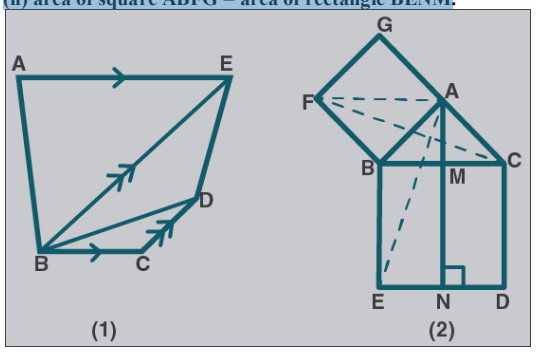# ML Aggarwal Solutions Class 9 Mathematics Solutions for Theorems on Area Exercise 14 in Chapter 14 - Theorems on Area

Question 17 Theorems on Area Exercise 14

(a) In the figure (1) given below, BC || AE and CD || BE. Prove that: area of

∆ABC= area of ∆EBD.

(b) In the figure (2) given below, ABC is a right-angled triangle at A. AGFB is a

square on the side AB and BCDE is a square on the hypotenuse BC. If AN ⊥ ED,

prove that:

(i) ∆BCF ≅ ∆ ABE.

(ii) area of square ABFG = area of rectangle BENM(a) Given:

From fig (1)

BC || AE and CD || BE

To prove:

area of ∆ABC= area of ∆EBD

Proof:

By joining CE.

We know that, from ∆ABC and ∆EBC

ar (∆ABC) = ar (∆EBC) ….. (1)

From EBC and ∆EBD

ar (∆EBC) = ar (∆EBD) …… (2)

From (1) and (2), we get

ar (∆ABC) = ar (∆EBD)

Hence proved.

(b) Given:

ABC is a right-angled triangle at A. Squares AGFB and BCDE is drawn on the side AB

and hypotenuse BC of ∆ABC. AN ⊥ ED which meets BC at M.

To prove:

(i) ∆BCF ≅ ∆ ABE.

(ii) area of square ABFG = area of rectangle BENM

From the figure (2)

(i) ∠FBC = ∠FBA + ∠ABC

So,

\begin{array}{l} \angle \mathrm{FBC}=90^{\circ}+\angle \mathrm{ABC} \ldots . .(1) \\ \angle \mathrm{ABE}=\angle \mathrm{EAC}+\angle \mathrm{ABC} \\ \mathrm{So}, \\ \angle \mathrm{ABE}=90^{\circ}+\angle \mathrm{ABC} \ldots \ldots(2) \end{array}

From (1) and (2), we get

∠FBC = ∠ABE ….. (3)

So, BC = BE

Now, in ∆BCF and ∆ABE

BF = AB

By using SAS axiom rule of congruency,

∴ ∆BCF ≅ ∆ ABE

Hence proved.

(ii) we know that,

∆BCF ≅ ∆ ABE

So, ar (∆BCF) = ar (∆ABE) ….. (4)

\begin{aligned} \angle \mathrm{BAG}+\angle \mathrm{BAC} &=90^{\circ}+90^{\circ} \\ &=180^{\circ} \end{aligned}

So, GAC is a straight line.

Now, from ∆BCF and square AGFB

ar (∆BCF) = ½ ar (square AGFB) …. (5)

From ∆ABE and rectangle BENM

ar (∆ABE) = ½ ar (rectangle BENM) ….. (6)

From (4), (5), and (6)

½ ar (square AGFB) = ½ ar (rectangle BENM)

ar (square AGFB) = ar (rectangle BENM)

Hence proved.

Related Questions
Exercises

Lido

Courses

Teachers

Book a Demo with us

Syllabus

Maths
CBSE
Maths
ICSE
Science
CBSE

Science
ICSE
English
CBSE
English
ICSE
Coding

Terms & Policies

Selina Question Bank

Maths
Physics
Biology

Allied Question Bank

Chemistry
Connect with us on social media!